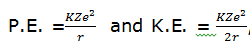# When a hydrogen atom is raised from the ground state to an excited state, (a) P.E decreases and K.E. increases (b)P.E. increases and K.E decreases (c)    Both K.E. and P.E. decrease (d)    Absorption spectrum9 years ago

(b), where, r is the radius of orbit which increases as we move from ground to an excited state. Therefore, when a hydrogen atom is raised from the ground state, it increases the value of r. As a result of this, P.E. increases (decreases in negative) and K.E. decreases.Kushagra Madhukar